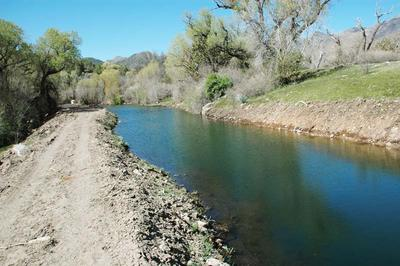online_fall_velocity:   Fall velocity of a spherical sediment particlew = {(4/3)[(gds)/CD][(γs - γ)/γ]}1/2

R = (w ds)/ν

Reference

 DESCRIPTION This program calculates the fall velocity [w] of a spherical sediment particle using an iterative procedure, with the following steps: The particle Reynolds number [R] is assumed equal to 100. For the given Reynolds number, the drag coefficient CD is obtained from Fig. 15-1 in the text, assuming a spherical particle. The fall velocity is calculated with Eq. 15-2, assuming γs = 2.65 (quartz particles). The particle Reynolds number is calculated with Eq. 15-3. The absolute difference between assumed and calculated Reynolds number is compared to a specified tolerance (0.0001). If the difference is greater than the tolerance, replace assumed with calculated value, and return to Step 2. Otherwise, stop and report results of last iteration. Output is the number of iterations, and the last drag coefficient, particle Reynolds number, and fall velocity (in m/s or fps) calculated. Default units is SI (metric). The program can take U.S. Customary units.

Thank you for running online_fall_velocity.   Please call again.